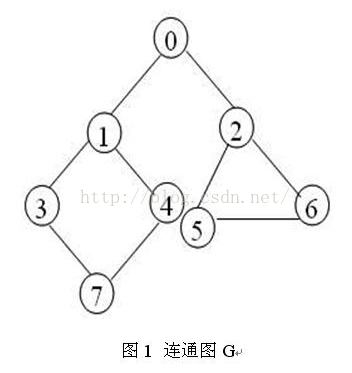# C++计算图任意两点间的所有路径

A 将始点标志位①置1，将其入栈

B 查看栈顶节点V在图中，有没有可以到达、且没有入栈、且没有从这个节点V出发访问过的节点

C 如果有，则将找到的这个节点入栈，这个顶点的标志位①置1，V的对应的此顶点的标志位②置1

D 如果没有，V出栈，并且将与v相邻的全部结点设为未访问，即全部的标志位②置0

E 当栈顶元素为终点时，设置终点没有被访问过，即①置0，打印栈中元素，弹出栈顶节点

F 重复执行B ? E,直到栈中元素为空1、 我们建立一个存储结点的栈结构，将起点3入栈，将结点3标记为入栈状态；
2、 从结点3出发，找到结点3的第一个非入栈没有访问过的邻结点1，将结点1标记为入栈状态，并且将3到1标记为已访问；
3、 从结点1出发，找到结点1的第一个非入栈没有访问过的邻结点0，将结点0标记为入栈状态，并且将1到0标记为已访问；
4、 从结点0出发，找到结点0的第一个非入栈没有访问过的邻结点2，将结点2标记为入栈状态，并且将0到2标记为已访问；
5、 从结点2出发，找到结点2的第一个非入栈没有访问过的邻结点5，将结点5标记为入栈状态，并且将2到5标记为已访问；
6、 从结点5出发，找到结点5的第一个非入栈没有访问过的邻结点6，将结点6标记为入栈状态，并且将5到6标记为已访问；
7、 栈顶结点6是终点，那么，我们就找到了一条起点到终点的路径，输出这条路径；
8、 从栈顶弹出结点6，将6标记为非入栈状态；
9、 现在栈顶结点为5，结点5没有非入栈并且非访问的结点，所以从栈顶将结点5弹出，并且将5到6标记为未访问；
10、        现在栈顶结点为2，结点2的相邻节点5已访问，6满足非入栈，非访问，那么我们将结点6入栈；
11、        现在栈顶为结点6，即找到了第二条路径，输出整个栈，即为第二条路径
12、        重复步骤8-11，就可以找到从起点3到终点6的所有路径；
13、        栈为空，算法结束。

```class Graph
{
private:
CArray<DataType,DataType> Vertices;
int Edge[MaxVertices][MaxVertices];
int numOfEdges;
public:
Graph();
~Graph();
void InsertVertex(DataType Vertex);
void InsertEdge(int v1,int v2,int weight);
int GetWeight(int i,int j);
int GetVertices();
DataType GetValue(int i);
};

```

```template<class T>
class Stack
{
private:
int m_size;
int m_maxsize;
T* data;
public:
Stack();
~Stack();
void push(T data); //压栈
T pop(); //出栈，并返回弹出的元素
T peek(); //查看栈顶元素
bool isEmpty(); //判断是否空
int getSize(); //得到栈的中元素个数
T* getPath(); //返回栈中所有元素
};
template<class T>
Stack<T>::Stack()
{
m_size=0;
m_maxsize=100;
data=new T[m_maxsize];
}
template<class T>
Stack<T>::~Stack()
{
delete []data;
}
template<class T>
T Stack<T>::pop()
{
m_size--;
return data[m_size];
}

template<class T>
void Stack<T>::push(T d)
{
if (m_size==m_maxsize)
{
m_maxsize=2*m_maxsize;
T* new_data=new T[m_maxsize];
for (int i=0;i<m_size;i++)
{
new_data[i]=data[i];
}
delete []data;
data=new_data;
}
data[m_size]=d;
m_size++;
}

template<class T>
T Stack<T>::peek()
{
return data[m_size-1];
}

template<class T>
bool Stack<T>::isEmpty()
{
if (m_size==0)
{
return TRUE;
}
else
{
return FALSE;
}
}

template<class T>
T* Stack<T>::getPath()
{
T* path=new T[m_size];
for (int i=0;i<m_size;i++)
{
path[i]=data[i];
}
return path;
}

template<class T>
int Stack<T>::getSize()
{
return m_size;
}
```

Vertex类，便于遍历全部的结点

```class CVertex
{
private:
int m_num;//保存与该顶点相邻的顶点个数
int *m_nei; //与该顶点相邻的顶点序号
int *m_flag; //与该顶点相邻的顶点是否访问过
bool isin; //该顶点是否入栈
public:
CVertex();
void Initialize(int num,int a[]);
int getOne(); //得到一个与该顶点相邻的顶点
void resetFlag(); //与该顶点相邻的顶点全被标记为未访问
void setIsin(bool);//标记该顶点是否入栈
bool isIn(); //判断该顶点是否入栈
void Reset();//将isin和所有flag置0
~CVertex();

};```
```CVertex::CVertex()
{
m_num=SIZE;
m_nei=new int[m_num];
m_flag=new int[m_num];
isin=false;
for (int i=0;i<m_num;i++)
{
m_flag[i]=0;
}

}
void CVertex::Initialize(int num,int a[])
{
m_num=num;
for (int i=0;i<m_num;i++)
{
m_nei[i]=a[i];
}
}
CVertex::~CVertex()
{
delete []m_nei;
delete []m_flag;
}
int CVertex::getOne()
{
int i=0;
for (i=0;i<m_num;i++)
{
if (m_flag[i]==0) //判断是否访问过
{
m_flag[i]=1; //表示这个顶点已经被访问，并将其返回
return m_nei[i];
}
}
return -1; //所有顶点都已访问过则返回-1
}
void CVertex::resetFlag()
{
for (int i=0;i<m_num;i++)
{
m_flag[i]=0;
}
}
void CVertex::setIsin(bool a)
{
isin=a;
}
bool CVertex::isIn()
{
return isin;
}
void CVertex::Reset()
{
for (int i=0;i<m_num;i++)
{
m_flag[i]=0;
}
isin=false;
}
```

```int a[SIZE],num;
for ( i=0;i<SIZE;i++)
{
num=0;
for (int j=0;j<SIZE;j++)
{

if (m_graph.Edge[i][j]!=MaxWeight&amp;&amp;i!=j)
{
a[num]=j;
num++;
}

}
vertex[i].Initialize(num,a);
```

```stack.push(selection1); //将起点压栈
vertex[selection1].setIsin(true); //标记为已入栈
int path_num=0;
while (!stack.isEmpty()) //判断栈是否空
{

int flag=vertex[stack.peek()].getOne(); //得到相邻的顶点
if (flag==-1) //如果相邻顶点全部访问过
{
int pop=stack.pop(); //栈弹出一个元素
vertex[pop].resetFlag(); //该顶点相邻的顶点标记为未访问
vertex[pop].setIsin(false); //该顶点标记为未入栈
continue; //取栈顶的相邻节点
}
if (vertex[flag].isIn()) //若已经在栈中，取下一个顶点
{
continue;
}
if (stack.getSize()>maxver-1) //判断栈中个数是否超过了用户要求的 ，这里是限制了一条路径节点的最大个数
{
int pop=stack.pop();
vertex[pop].resetFlag();
vertex[pop].setIsin(false);
continue;
}
stack.push(flag); //将该顶点入栈

vertex[flag].setIsin(true); //记为已入栈

if (stack.peek()==selection2) //如果栈顶已经为所求，将此路径记录
{
int *path=stack.getPath();
//保存路径的代码省略
int pop=stack.pop(); //将其弹出，继续探索
vertex[pop].setIsin(false); //清空入栈的标志位
}

}
```